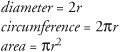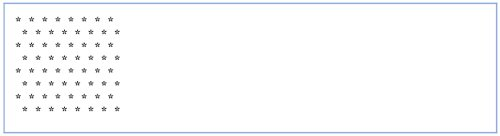# Wrap-Up

### Answers to Self Review Exercises

 20.1 a) init, start, paint. b) stop. c) JApplet (or Applet). d) appletviewer. e) start. f) HTML. g) init. h) paint. i) destroy. j) ,.

### Exercises

 20.2 Write an applet that asks the user to enter two floating-point numbers, obtains the two numbers from the user and draws their sum, product (multiplication), difference and quotient (division). Use the techniques shown in Fig. 20.10. 20.3 Write an applet that asks the user to enter two floating-point numbers, obtains the numbers from the user and displays the two numbers first and then the larger number followed by the words "is larger" as a string on the applet. If the numbers are equal, the applet should print the message "These numbers are equal". Use the techniques shown in Fig. 20.10. 20.4 Write an applet that inputs three floating-point numbers from the user and displays the sum, average, product, smallest and largest of these numbers as strings on the applet. Use the techniques shown in Fig. 20.10. 20.5 Write an applet that asks the user to input the radius of a circle as a floating-point number and draws the circle's diameter, circumference and area. Use the value 3.14159 for p. Use the techniques shown in Fig. 20.10. [Note: You may also use the predefined constant Math.PI for the value of p. This constant is more precise than the value 3.14159. Class Math is defined in the java.lang package, so you do not need to import it.] Use the following formulas (r is the radius):20.6 Write an applet that reads five integers, determines which are the largest and smallest integers in the group and prints them. Use only the programming techniques you learned in this chapter and Chapter 2. Draw the results on the applet. 20.7 Write an applet that draws a checkerboard pattern as follows:20.8 Write an applet that draws rectangles of different sizes and locations. 20.9 Write an applet that allows the user to input values for the arguments required by method drawRect, then draws a rectangle using the four input values. 20.10 Class Graphics contains method drawOval, which takes as arguments the same four arguments as method drawRect. The arguments for method drawOval specify the "bounding box for the ovalthe sides of the bounding box are the boundaries of the oval. Write a Java applet that draws an oval and a rectangle with the same four arguments. The oval will touch the rectangle at the center of each side. 20.11 Modify the solution to Exercise 20.10 to output ovals of different shapes and sizes. 20.12 Write an applet that allows the user to input the four arguments required by method drawOval, then draws an oval using the four input values.Java How to Program (6th Edition) (How to Program (Deitel))
ISBN: 0131483986
EAN: 2147483647
Year: 2003
Pages: 615

Similar book on Amazon